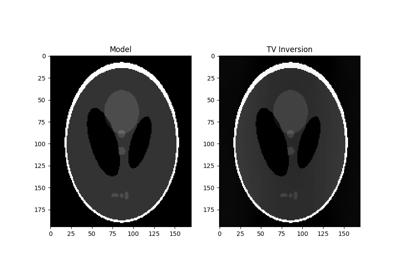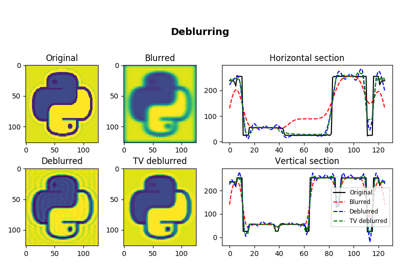# pylops.optimization.sparsity.SplitBregman¶

pylops.optimization.sparsity.SplitBregman(Op, RegsL1, data, niter_outer=3, niter_inner=5, RegsL2=None, dataregsL2=None, mu=1.0, epsRL1s=None, epsRL2s=None, tol=1e-10, tau=1.0, x0=None, restart=False, show=False, **kwargs_lsqr)[source]

Split Bregman for mixed L2-L1 norms.

Solve an unconstrained system of equations with mixed L2-L1 regularization terms given the operator Op, a list of L1 regularization terms RegsL1, and an optional list of L2 regularization terms RegsL2.

Parameters: Op : pylops.LinearOperator Operator to invert RegsL1 : list L1 regularization operators data : numpy.ndarray Data niter_outer : int Number of iterations of outer loop niter_inner : int Number of iterations of inner loop RegsL2 : list Additional L2 regularization operators (if None, L2 regularization is not added to the problem) dataregsL2 : list, optional L2 Regularization data (must have the same number of elements of RegsL2 or equal to None to use a zero data for every regularization operator in RegsL2) mu : float, optional Data term damping epsRL1s : list L1 Regularization dampings (must have the same number of elements as RegsL1) epsRL2s : list L2 Regularization dampings (must have the same number of elements as RegsL2) tol : float, optional Tolerance. Stop outer iterations if difference between inverted model at subsequent iterations is smaller than tol tau : float, optional Scaling factor in the Bregman update (must be close to 1) x0 : numpy.ndarray, optional Initial guess restart : bool, optional The unconstrained inverse problem in inner loop is initialized with the initial guess (True) or with the last estimate (False) show : bool, optional Display iterations log **kwargs_lsqr Arbitrary keyword arguments for scipy.sparse.linalg.lsqr solver xinv : numpy.ndarray Inverted model itn_out : int Iteration number of outer loop upon termination

Notes

Solve the following system of unconstrained, regularized equations given the operator $$\mathbf{Op}$$ and a set of mixed norm (L2 and L1) regularization terms $$\mathbf{R_{L2,i}}$$ and $$\mathbf{R_{L1,i}}$$, respectively:

$J = \mu/2 ||\textbf{d} - \textbf{Op} \textbf{x} |||_2 + \sum_i \epsilon_{{R}_{L2,i}} ||\mathbf{d_{{R}_{L2,i}}} - \mathbf{R_{L2,i}} \textbf{x} |||_2 + \sum_i || \mathbf{R_{L1,i}} \textbf{x} |||_1$

where $$\mu$$ and $$\epsilon_{{R}_{L2,i}}$$ are the damping factors used to weight the different terms of the cost function.

The generalized Split Bergman algorithm is used to solve such cost function: the algorithm is composed of a sequence of unconstrained inverse problems and Bregman updates. Note that the L1 terms are not weighted in the original cost function but are first converted into constraints and then re-inserted in the cost function with Lagrange multipliers $$\epsilon_{{R}_{L1,i}}$$, which effectively act as damping factors for those terms. See  for detailed derivation.

The scipy.sparse.linalg.lsqr solver and a fast shrinkage algorithm are used within the inner loop to solve the unconstrained inverse problem, and the same procedure is repeated niter_outer times until convergence.

  Goldstein T. and Osher S., “The Split Bregman Method for L1-Regularized Problems”, SIAM J. on Scientific Computing, vol. 2(2), pp. 323-343. 2008.

## Examples using pylops.optimization.sparsity.SplitBregman¶Total Variation (TV) Regularization04. Image deblurring